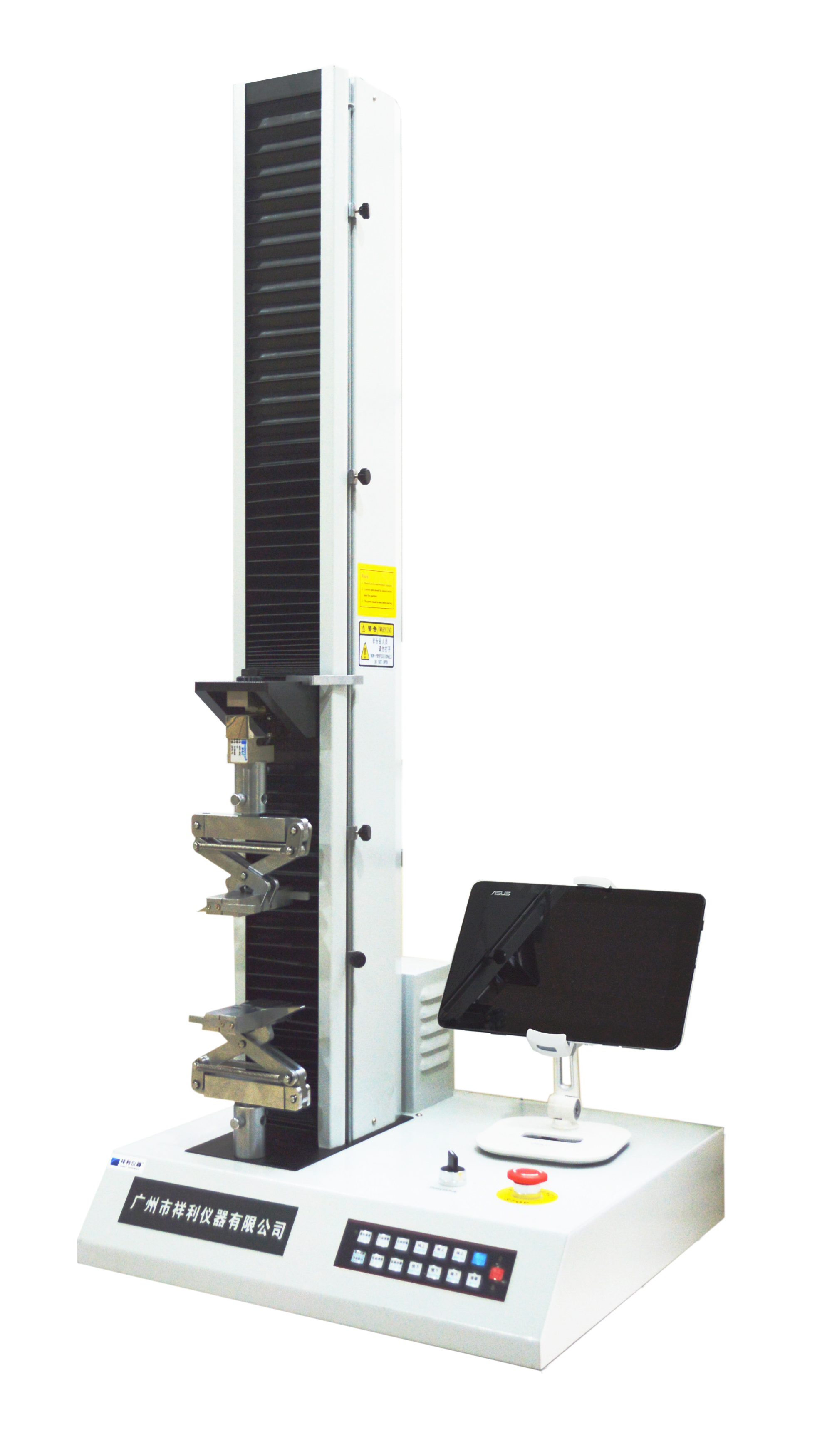# Tensile machine test knowledgeWhen using the tensile testing machine, we will find that the impact of the tensile testing machine will vary with the material. Therefore, the tensile test must be carried out in strict accordance with the requirements specified by the standard test method.

1. Tensile strength: The tensile strength increases with the increase of the test speed, but tends to be stable after reaching a certain stage.

2. Yield strength: When the test speed is slow, the difference between the yield strength and the tensile strength is relatively large; the faster the test speed, the difference between the yield strength and the tensile strength is gradually reduced.

3. Elongation after fracture: The increase of tensile speed reduces the elongation after fracture, and the elongation decreases after the break to a certain stage. (In addition, the large plastic tensile strength and the elongation after break are sensitive to the tensile speed, while the small plastic tensile strength and the post-break elongation are relatively less sensitive to the tensile speed.

In general, the change of the tensile speed has the same effect on the test results as above, but for the plastic material, it belongs to the viscoelastic material, and its stress relaxation process is closely linked with the deformation speed. When the tensile speed decreases, the tensile strength decreases and the elongation at break increases. When the tensile speed increases, the plastic exhibits brittleness, tensile strength increases, and elongation at break decreases the simply supported beam impact tester.

Get the latest price? We'll respond as soon as possible(within 12 hours)